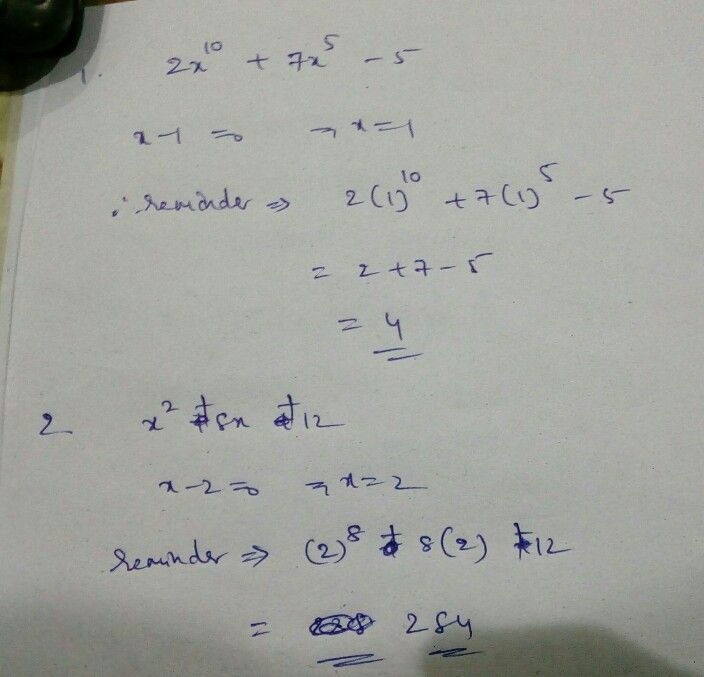Symbol
Problem$A$ Answer the $fo1ossing$ $1$ What is the remainder when $2x^{10+7x^{5}}$ $-51$ is divided $byx-17$ $2$ What is the remainder $x2+8x+12$ when divided $byx-22$ $3.$ What are the factors of $x^{2+8x+122}$ $4$ If the remainder of the $x3-kx2+3x-2$ when divided $byx-1$ is $4$ find the value ofk. $B$ $Ansscring$ the following questions. $1$ How do you factor polynomials? Discuss the mathematics concepts and principles applied when factoring polynomials. $2$ What new realizations do you have about factoring polynomials? How would you connect this to real life? How would you use this in making decisions?
10th-13th grade
Other
SolutionQanda teacher - premsagar